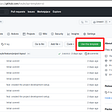# LDPC — low density parity check code

5G NR uses LDPC for channel coding on the traffic channel. LDPC corrects channel errors by maintaining parity bits for a selection of the data bits. The figure below shows the relation between the data and parity bits.

Most data bits are backed by multiple parity bits. When a parity check failure is detected, information from the multiple parity bits can be used to retrieve the original data bit.

# Intuitive understanding of LDPC

The following video explains LDPC from first principles. We recommend watching this video before reading the rest of the blog post.

# Block code basics

Consider a parity based code that operates on a block on `n` bits. Out of the `n` bits in the block, `k` bits carry data and `r` bits carry parity. Thus

`n = k + r`

Now consider a code with a 7-bit block length with 3 parity bits per block. This means:

`n = 7, k = 4, r = 3`

This code with be considered a rate 4/7 code as there are 4 bits of data for every 7 bits of transmission.

If the 7 bits of the block are labeled `c1` to `c7`. Here `c1`, `c2`, `c3` and `c4` are data bits. `c5`, `c6` and `c7` are parity bits. We can define the parity constraints as shown below. The constraints are defined with ⊕ modulo 2 additions (essentially an exclusive-or operation).

`c1 ⊕ c2 ⊕ c3 ⊕ c5 = 0              e1c1 ⊕ c2 ⊕ c4 ⊕ c6 = 0              e2c1 ⊕ c3 ⊕ c4 ⊕ c7 = 0              e3`

The above equation can also be represented in a matrix form. Each equation maps to a row in the matrix. 0 terms have been introduced for columns that did not exist in the original equation.

`c1  c2  c3  c4  c5  c6  c71   1   1   0   1   0   01   1   0   1   0   1   01   0   1   1   0   0   1`

The equations described above are typically represented as a Tanner graph. The data and parity bits are shown on the top. The equations placing constraints on the data and parity bits are represented below with connecting lines to the bits covered by each constraint.

# Encoding

Let’s assume that the user data to send is `1001`.

`c1  c2  c3  c41   0   0   1`

We apply this to our parity equations.

`1 ⊕ 0 ⊕ 0 ⊕ c5 = 01 ⊕ 0 ⊕ 1 ⊕ c6 = 01 ⊕ 0 ⊕ 1 ⊕ c7 = 0`

The parity bits `c5`, `c6` and `c7` must be assigned values so that the above equations are satisfied. The equations will be satisfied when even number of bits are set in each equation.

`1 ⊕ 0 ⊕ 0 ⊕ 1 = 01 ⊕ 0 ⊕ 1 ⊕ 0 = 01 ⊕ 0 ⊕ 1 ⊕ 0 = 0`

This results in the following seven bits.

`c1  c2  c3  c4  c5  c6  c71   0   0   1   1   0   0`

The Tanner graph for the data bits and the constraint nodes is shown below.

# Decoding

## Bit flipping algorithm

Let’s assume that `c3` bit is received in error at the receiver (orange node in the top row). This error results in the two parity check equation failures (orange nodes in the bottom row).

Here we can apply the simplest LDPC decoding algorithm:

Here the `c3` bit is getting two negative votes from the parity check equations. There are no positive votes, so `c3` flips itself. Flipping the bit results in two positive votes to `c3`. At this point all parity checks are passing, thus completing the decode.

## Message passing algorithm

Bit flipping algorithm is simple but does not have great error detection properties. Message passing algorithms improve the decoder performance by iteratively exchanging estimates between the data bit nodes and the parity check nodes. The scheme is described in the following presentation.

The iterative nature of LDPC is visually illustrated by the following short video. The video shows rate 1/2 code iterations for the transmission of an image. Each iteration recovers more of the image. The iterations proceed until the full image is recovered.

## Belief propagation decoder

This scheme used belief propagation techniques that were developed for iterating in neural networks. Belief propagation algorithms offer the best LDPC decode performance. They are introduced in the following paper.

# Rate matching and HARQ support with LDPC

5G NR has unique requirements for supporting incremental redundancy via HARQ. In addition to this, rate matching needs to be supported to dynamically adjust the coding to the allocated resources.

3GPP has standardized on QC-LDPC (Quasi Cyclic LDPC) for HARQ and rate matching support. Details about the scheme are available in the following patent application.

--

--

--

## Towards Understanding Grover’s Search Algorithm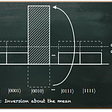## A Million Dollar Question in the era of Deep Learning## LINEAR ALGEBRA FOR DATA SCIENCE AND MACHINE LEARNING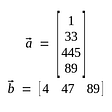## Scaling up running Keras models on ML Engine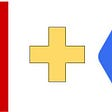## Implementation Of KNN (From Scratch in PYTHON)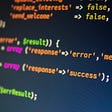## Viewing the E.Coli imbalance dataset in 3D with Python## Seq2Seq with Pytorch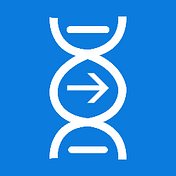## EventHelix

@EventHelix — 5G | LTE | Networking

## Building a serverless application using step functions, API Gateway, Lambda and S3 in AWS.## Configuring infinispan persistence for a table with MySQL as database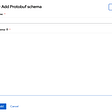## A JSON End-Point for shield.io badge